### Example 2.4 Linear Assignment Problem, Sparse Format versus Dense Format

This example looks at the problem of assigning swimmers to strokes based on their best times. However, in this case certain swimmers are not eligible to perform certain strokes. A missing (.) value in the data matrix identifies an ineligible assignment. For example:

data RelayTimesMatrix;
input name $sex$ back breast fly free;
datalines;
Sue     F   .  36.7 28.3 36.1
Karen   F 34.6    .    . 26.2
Jan     F 31.3    . 27.1    .
Andrea  F 28.6    . 29.1    .
Carol   F 32.9    . 26.6    .
;


Recall that the linear assignment problem can also be interpreted as the minimum-weight matching in a bipartite graph. The eligible assignments define links between the rows (swimmers) and the columns (strokes), as in Output 2.4.1.

Output 2.4.1: Bipartite Graph for Linear Assignment Problem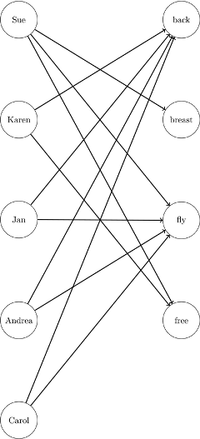Because of this, you can represent the same data in RelayTimesMatrix with a links data set as follows:

data RelayTimesLinks;
input name $attr$ cost;
datalines;
Sue     breast 36.7
Sue     fly    28.3
Sue     free   36.1
Karen   back   34.6
Karen   free   26.2
Jan     back   31.3
Jan     fly    27.1
Andrea  back   28.6
Andrea  fly    29.1
Carol   back   32.9
Carol   fly    26.6
;


This graph must be bipartite (such that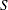and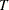are disjoint); if it is not, PROC OPTNET returns an error.

Now, you can use either input format to solve the same problem as follows:

proc optnet
data_matrix = RelayTimesMatrix;
linear_assignment
out      = LinearAssignMatrix
weight   = (back--free)
id       = (name sex);
run;

proc optnet
graph_direction = directed
from         = name
to           = attr
weight       = cost;
linear_assignment
run;


When you use the graph input format, the LINEAR_ASSIGNMENT options WEIGHT= and ID= are not used directly.

The data sets LinearAssignMatrix and LinearAssignLinks now contain the optimal assignments, as shown in Output 2.4.2 and Output 2.4.3.

Output 2.4.2: Optimal Assignments for Swim Times (Dense Input)

name sex assign cost
Sue F breast 36.7
Karen F free 26.2
Andrea F back 28.6
Carol F fly 26.6
118.1

Output 2.4.3: Optimal Assignments for Swim Times (Sparse Input)

name attr cost
Sue breast 36.7
Karen free 26.2
Andrea back 28.6
Carol fly 26.6
118.1

The optimal assignments are shown graphically in Output 2.4.4.

Output 2.4.4: Optimal Assignments for Swim TimesFor large problems where a number of links are forbidden, the sparse format can be faster and can save a great deal of memory. Consider an example that uses the format of the DATA_MATRIX= option with 15,000 columns (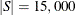) and 4,000 rows (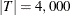). To store the dense matrix in memory, PROC OPTNET needs to allocate approximately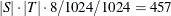MB. If the data have mostly ineligible links, then the sparse (graph) format that uses the DATA_LINKS= option is much more efficient with respect to memory. For example, if the data have only 5% of the eligible links (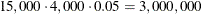), then the dense storage would still need 457 MB, while the sparse storage needs approximately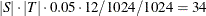MB. If the problem is fully dense (all links are eligible), then the dense format that uses the DATA_MATRIX= option is the most efficient.